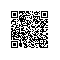# python 循环

循环

>>> 1 + 2 + 3
6

Python的循环有两种，一种是for...in循环，依次把list或tuple中的每个元素迭代出来，看例子：

names = ['Michael', 'Bob', 'Tracy']
for name in names:
print(name)

Michael
Bob
Tracy

sum = 0
for x in [1, 2, 3, 4, 5, 6, 7, 8, 9, 10]:
sum = sum + x
print(sum)

>>> list(range(5))
[0, 1, 2, 3, 4]
range(101)就可以生成0-100的整数序列，计算如下：

# -*- coding: utf-8 -*-

sum = 0
for x in range(101):
sum = sum + x
print(sum)

sum = 0
n = 99
while n > 0:
sum = sum + n
n = n - 2
print(sum)使用钉钉扫一扫加入圈子
+ 订阅